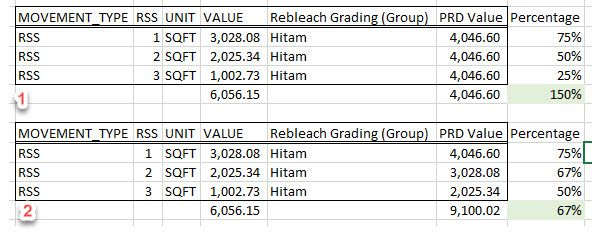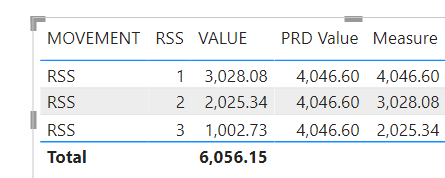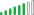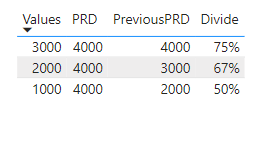cancel
Showing results for
Did you mean:

Fabric is Generally Available. Browse Fabric Presentations. Work towards your Fabric certification with the Cloud Skills Challenge.Helper IV

## Return values from previous parameters

Hi expert,

I have an issue with one of my report. Currently what I am doing in my report is like in table (1) below. I divided all RSS Value with PRD value (4,046.60).

But, what I really want is like in table (2) to get my desired percentage:
if RSS=1, then it will return PRD Value, 4,046.60
else if RSS= 2, then it will return Value of RSS 1 which is 3,028.08
else if RSS= 3, then it will return Value of RSS 2 which is 2,025.34

*RSS column is whole number datatypeBig thanks for your help 🤝

1 ACCEPTED SOLUTIONCommunity Support

Hi, @New_be

You can try the following methods.

``````Measure =
returnIn order to get Total to output the correct value, the IF(HASONEVALUE()) function is also required.

``````Pro = IF(HASONEVALUE('Table'[RSS]),[Measure],
``Rercentage = DIVIDE(SUM('Table'[VALUE]),[Pro])``Does this match the output you expect?

Best Regards,

Community Support Team _Charlotte

If this post helps, then please consider Accept it as the solution to help the other members find it more quickly.

4 REPLIES 4Community Support

Hi, @New_be

You can try the following methods.

``````Measure =
returnIn order to get Total to output the correct value, the IF(HASONEVALUE()) function is also required.

``````Pro = IF(HASONEVALUE('Table'[RSS]),[Measure],
``Rercentage = DIVIDE(SUM('Table'[VALUE]),[Pro])``Does this match the output you expect?

Best Regards,

Community Support Team _Charlotte

If this post helps, then please consider Accept it as the solution to help the other members find it more quickly.Helper IV

Thanks! it works!Solution Supplier

Hi @New_be

First create a calculated column with the following code:

``Rank = RANKX(ALL(PRD),'PRD'[RSS],,ASC,Dense)``

Then create another calculated column:

``````PreviousPRD =
VAR CurrentIndex = 'PRD'[Rank]
VAR PreviousIndex = CALCULATE(MAX('PRD'[Rank]),FILTER('PRD','PRD'[Rank] < CurrentIndex),ALL('PRD'[Rank]))
VAR Result = CALCULATE(MAX('PRD'[Values]),FILTER('PRD','PRD'[Rank] = PreviousIndex))
return IF(Result = BLANK(),FIRSTNONBLANKVALUE('PRD'[PRD],'PRD'[PRD]),Result)``````

Finally create a 3rd calculated column

``Divide = DIVIDE('PRD'[Values],'PRD'[PreviousPRD])``

Result:When you add the Divide column, don't forget to change the data format to percentage.

Kind regards,
Seanan
If this post helped, please consider accepting it as the solution.Helper IVAnnouncements#### Power BI Monthly Update - November 2023

Check out the November 2023 Power BI update to learn about new features.#### Fabric Community News unified experience

Read the latest Fabric Community announcements, including updates on Power BI, Synapse, Data Factory and Data Activator.#### The largest Power BI and Fabric virtual conference

130+ sessions, 130+ speakers, Product managers, MVPs, and experts. All about Power BI and Fabric. Attend online or watch the recordings.Top Solution Authors
Top Kudoed Authors
Users online (2,000)